Question

a. Compute the total stiffness matrix [K] of the assemblage shown in Figure 3-1 by superimposing...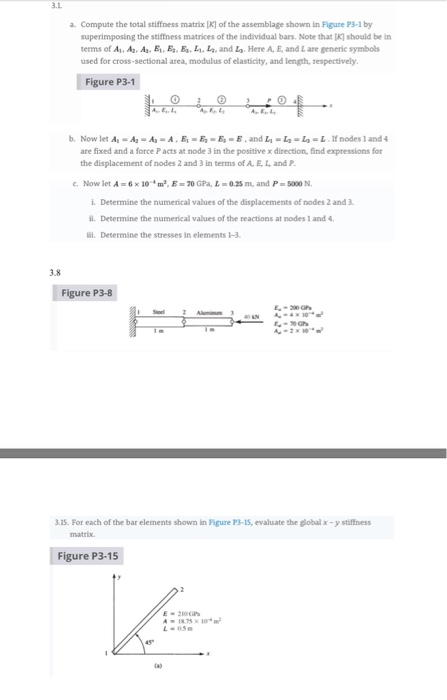a. Compute the total stiffness matrix [K] of the assemblage shown in Figure 3-1 by superimposing the stiffness matrices of the individual bars. Note that should be in terms of A. As, A, E, E E, L. and L. Here A, E, and are generic symbols used for cross-sectional area modulus of elasticity, and length, respectively Figure P3-1 Now let As - Ag-A-A.E E, E E and L-L L -L nodes 1 and 4 are fixed and a force Pacts at mode 3 in the positive x direction, find expressions for the displacement of nodes 2 and 3 in terms of A E L and Now let A = 6x 10 = 70 GPL = 0.25 m, and P-5000 N Determine the numerical values of the displacements of modes 2 and 3 ll. Determine the numerical values of the reactions at nodes 1 and 4 l. Determine the stresses in elements 1-3. Figure P3-8 3.15. For each of the bar elements shown in Figure 13-15, evaluate the globalxy stiffness Figure P3-15 /9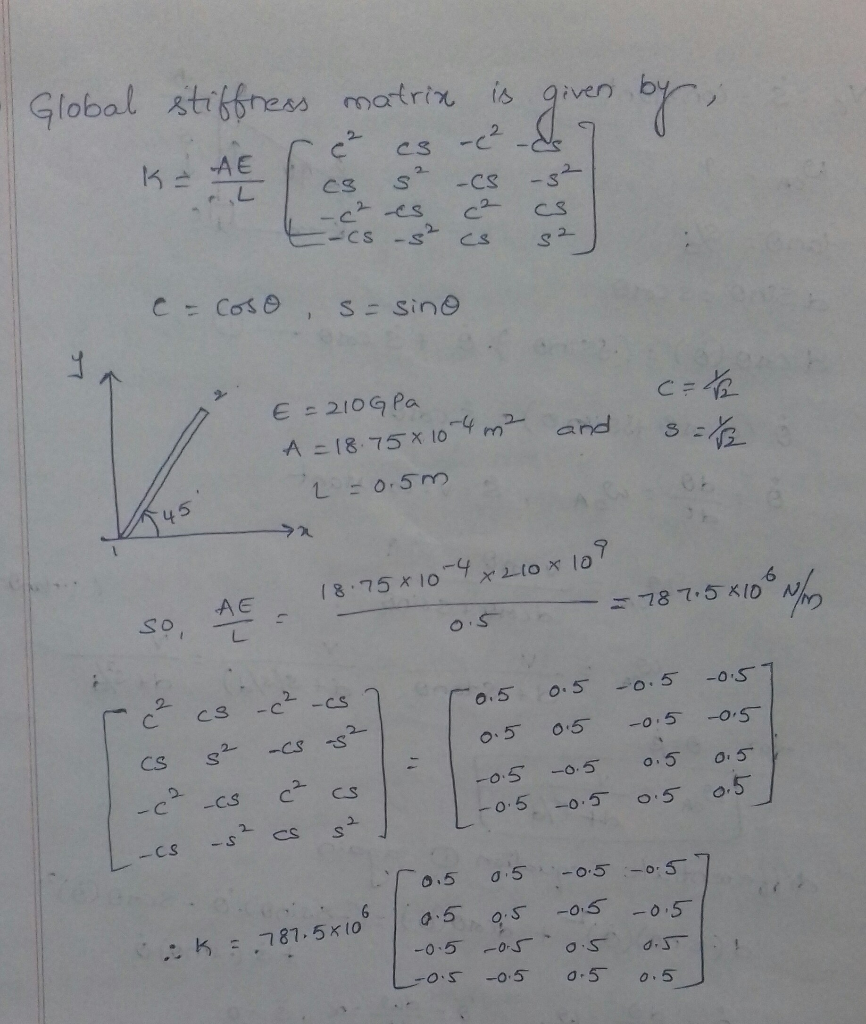Earn Coins

Coins can be redeemed for fabulous gifts.

Similar Homework Help Questions
• For the truss shown in the below figure, determine the stifness matrix for each truss element,...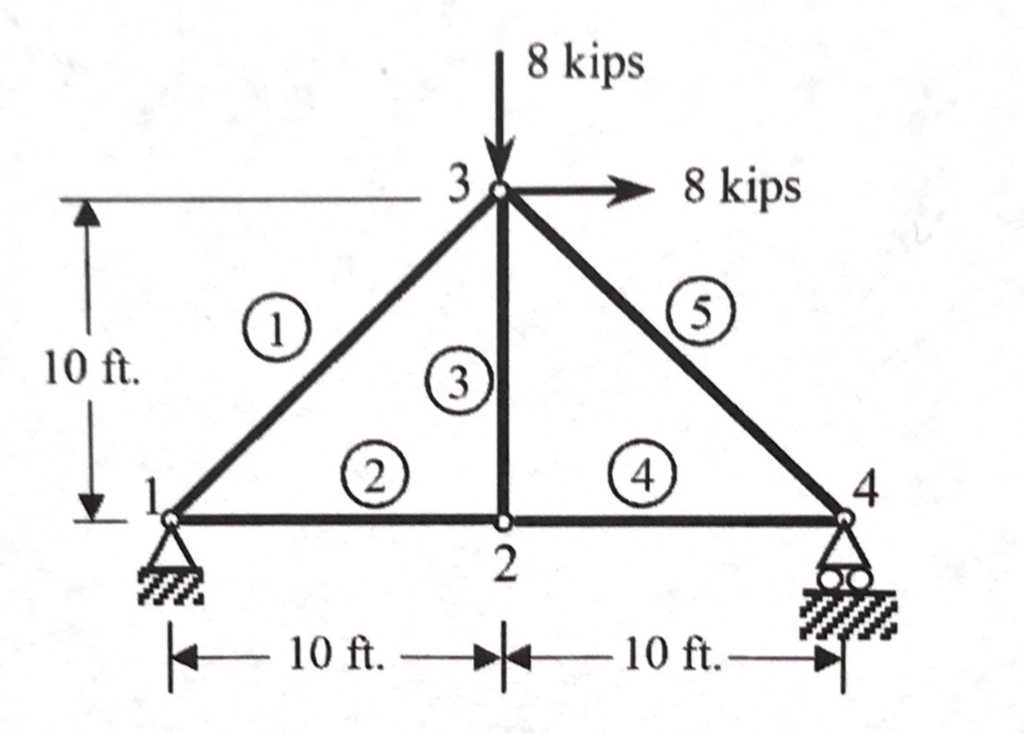For the truss shown in the below figure, determine the stifness matrix for each truss element, the stiffness matrix for entire truss, the displacements at nodes 1 through 4, and the force in elements 1 through 5. Also, determine the force in each element. Let A = 3 in2, E = 30 x 106 psi for all elements. 8 kips 8 kips 10 ft. 3 4 2 トー-10ft.-*-10 ft.

• N is 1 QUESTION 2 For the bar assemblage shown Data: in the figure, determine the...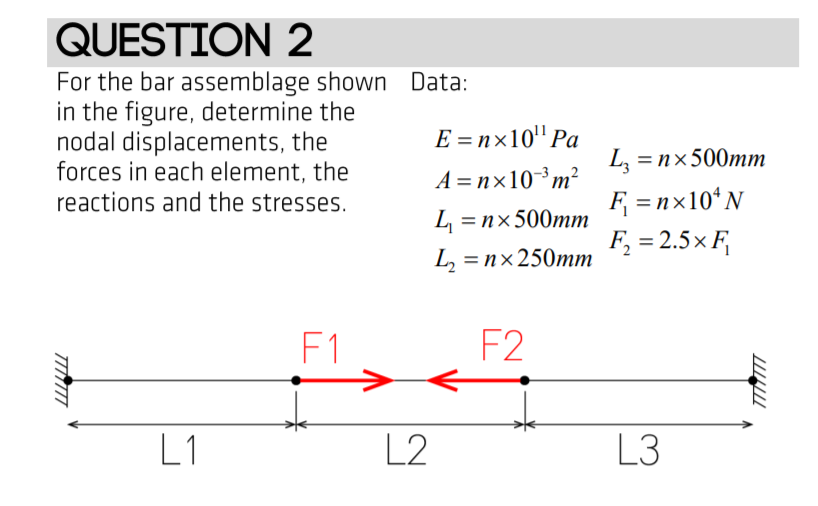N is 1 QUESTION 2 For the bar assemblage shown Data: in the figure, determine the nodal displacements, the E =nx10" Pa forces in each element, the A=nx10-?m? reactions and the stresses. L =nx 500mm Ly =n x500mm F=nx104N L2 =nx 250mm F, = 2.5x F, MITT L 1 L2

• Given the indeterminate beam shown below, use FEM to compute the final stiffness matrix and force...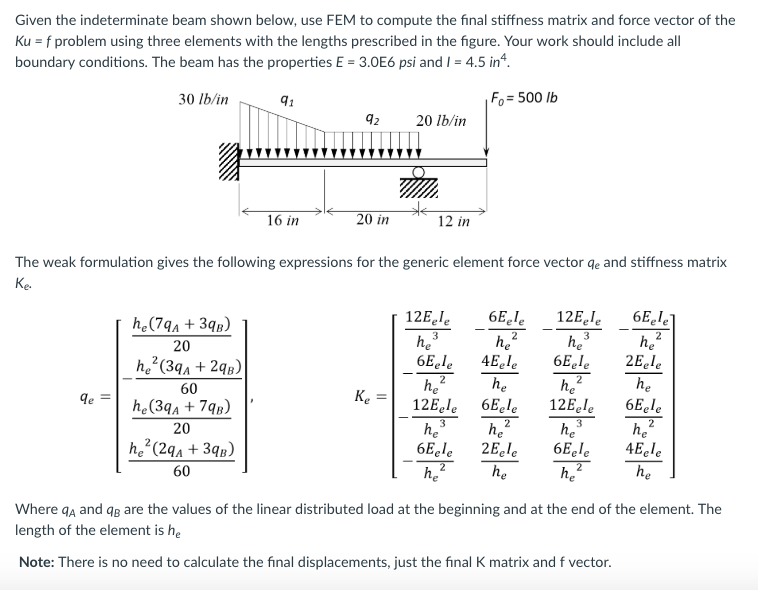Given the indeterminate beam shown below, use FEM to compute the final stiffness matrix and force vector of the Ku f problem using three elements with the lengths prescribed in the figure. Your work should include all boundary conditions. The beam has the properties E 3.0E6 psi and I 4.5 in 30 lb/in Fo-500 q2 20 lb/in 12 in The weak formulation gives the following expressions for the generic element force vector qe and stiffness matrix Ke. 12Eele 6Eel 20...

• Finite element problems For the bar elements shown in Figure P3–16, the global displacement have been...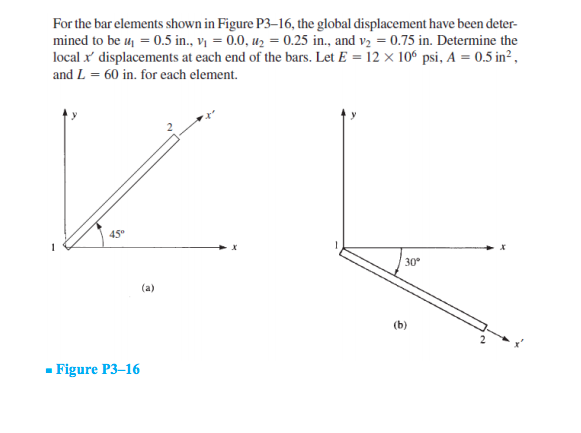Finite element problems For the bar elements shown in Figure P3–16, the global displacement have been deter- mined to be up = 0.5 in., V = 0.0, uy = 0.25 in., and V2 = 0.75 in. Determine the local x' displacements at each end of the bars. Let E = 12 x 106 psi, A = 0.5 in?, and L = 60 in. for each element. 45° 30° (a) (b) - Figure P3–16

• 2 k3 2 3 4 a. (10 points) Obtain the global stiffness matrix K using direct...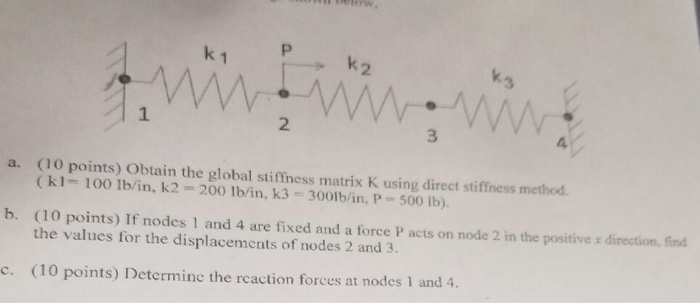2 k3 2 3 4 a. (10 points) Obtain the global stiffness matrix K using direct stiffness method (k1- 100 1b/in, k2 200 lb/in, k3 3001b/in, P-500 Ib). (10 points) If nodes 1 and 4 are fixed and a force P acts on node 2 in the positive x direction, fin the values for the displacements of nodes 2 and 3. b. c. (10 points) Deter odes 1 and 4

• Solve all problems using the finite element stiffness method. For the rigid frame shown in Figure P5-4, determine (1) the nodal displacements and rotation at node 4, (2) the reactions, and (3) the fo...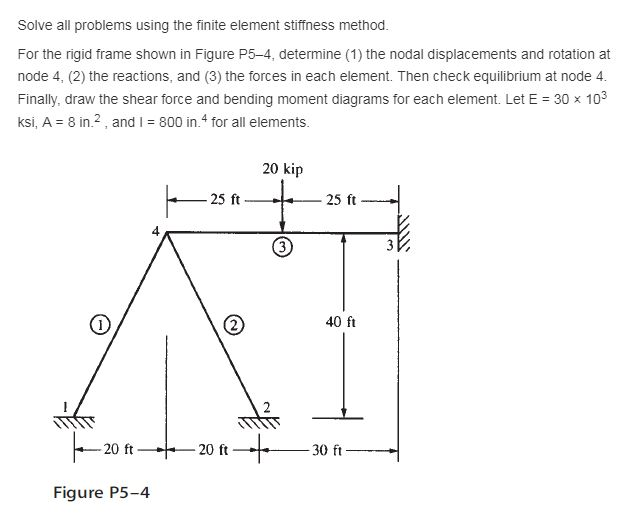Solve all problems using the finite element stiffness method. For the rigid frame shown in Figure P5-4, determine (1) the nodal displacements and rotation at node 4, (2) the reactions, and (3) the forces in each element. Then check equilibrium at node 4 Finally, draw the shear force and bending moment diagrams for each element. Let E 30x 103 ksi, A 8 in2, and I 800 in.4 for all elements. 20 kip 25 ft 25 ft 40 ft Figure P5-4...

• For the system shown below, (a) the global stiffness matrix   (b)displacements of nodes 2 and 3...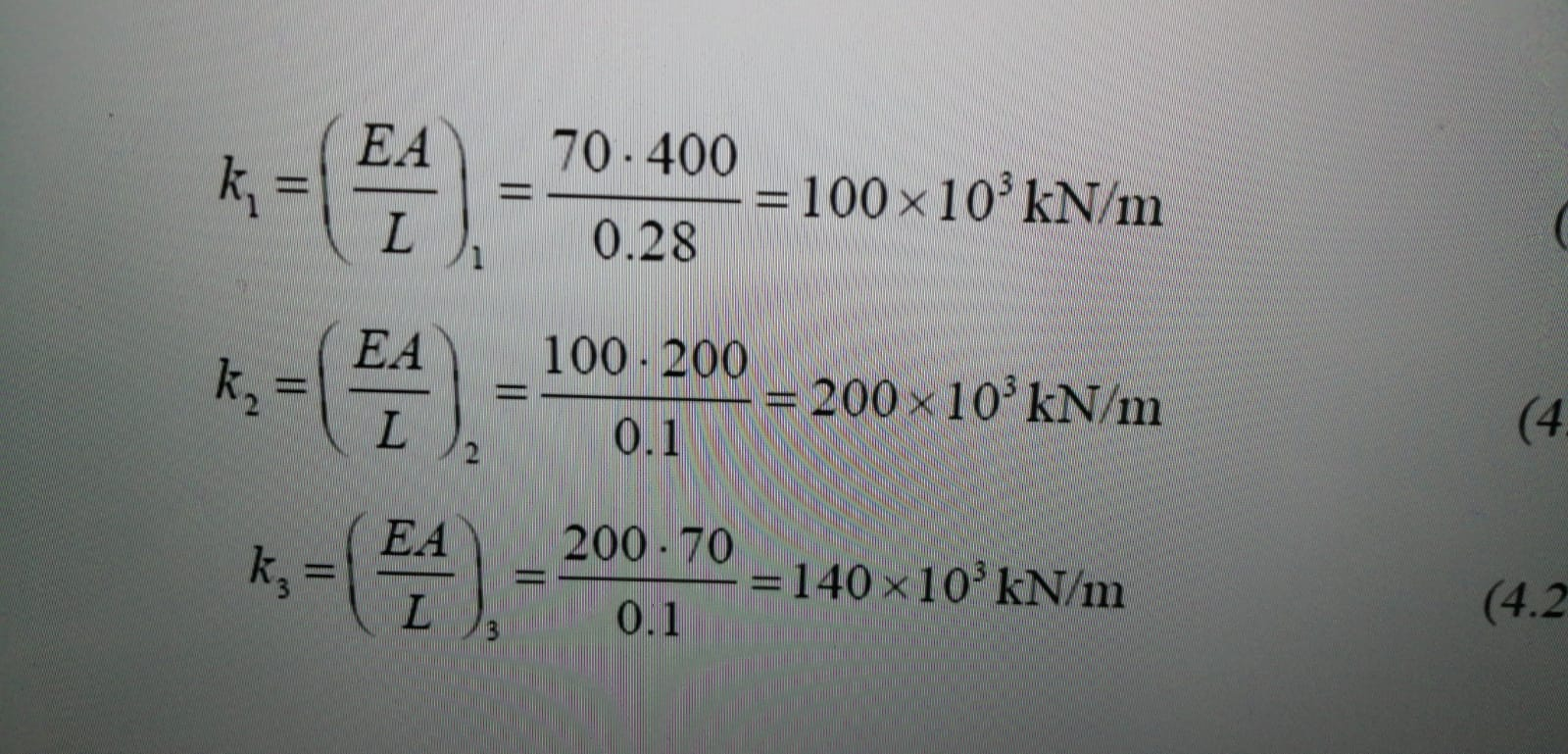For the system shown below, (a) the global stiffness matrix   (b)displacements of nodes 2 and 3 (c)the reaction forces at nodes 1 and 4 (d)the force in the members EA TRATAMI 70-400 = 100 x 10 kN/m 0.28 ATT L ( EA k, 100 - 200 = 200 x 10 kN/m 0.1 L (4 EA k, 200.70 =140x10 kN/m 0.1 I (4.2 X tretiet 0.28 2 vyos Imool

• Problem 2 135 pts The grid shown in figure 2 is rigidly fixed at nodes 2...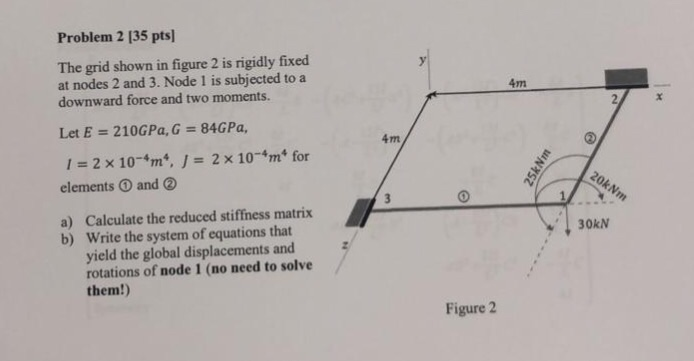Problem 2 135 pts The grid shown in figure 2 is rigidly fixed at nodes 2 and 3. Node 1 is subjected to a downward force and two moments. 4m Let E = 210GPa, G = 84GPa, 4m 1-2 × 10-4m", J = 2 × 10-4m" for elements ① and ② Calculate the reduced stiffness matrix Write the system of equations that yield the global displacements and rotations of node 1 (no need to solve them!) a) b) 30kN Figure...

• 1. (60%) For the truss system shown below (a) (12%) Determine the element stiffness matrix w.r.t....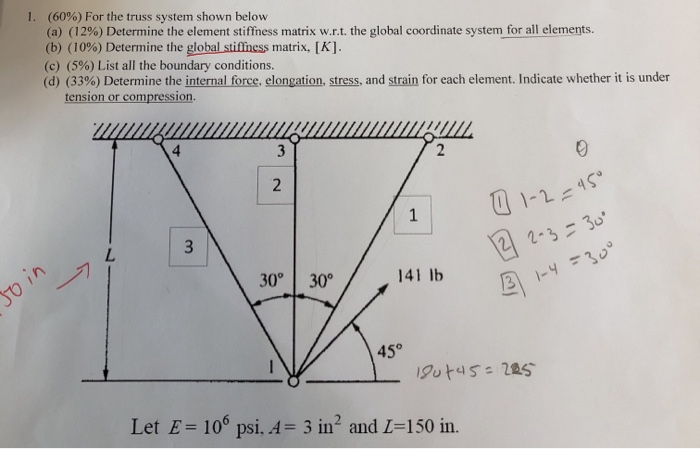1. (60%) For the truss system shown below (a) (12%) Determine the element stiffness matrix w.r.t. the global coordinate system for all elements. (b) (10%) Determine the global stiffness matrix, [K]. (c) (5%) List all the boundary conditions. (d) (33%) Determine the internal force, elongation, stress, and strain for each element. Indicate whether it is under tension or compression. My LLLLLLLL 1 0 1-2=45° \ 30° 30° / 14116 141 16 12 2-3 = 30 3 1-4=300 Join But 45=225...

• For the beam elements shown (Node 1 is fixed and node 3 is a pin); the...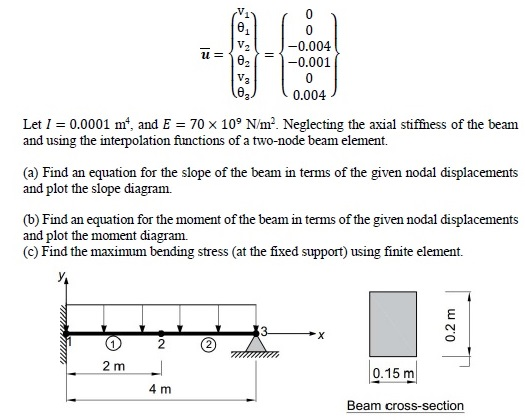For the beam elements shown (Node 1 is fixed and node 3 is a pin); the nodal displacements have been calculated in meters and radians as: V. V2 -0.001 V. 0.004 Let l 0.0001 m. and E 70 109 Nm: Neglecting the axial stiffness of the beam and using the interctions of a two-node beam element. (a) Find an equation for the slope of the beam in terms of the given nodal displacements and plot the slope diagram. (b) Find...